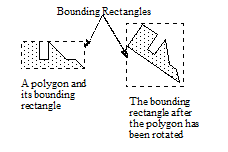### 2.5.7 Bounding Rectangles

Every bounded region in CLIM has a derived bounding rectangle , which is the smallest rectangle that contains every point in the region and which may contain additional points as well. Unbounded regions do not have any bounding rectangle. For example, all windows and output records have bounding rectangles whose coordinates are relative to the bounding rectangle of the parent of the window or output record.

The coordinate system in which the bounding rectangle is maintained depends on the context. For example, the coordinates of the bounding rectangle of a sheet are expressed in the sheet's parent's coordinate system. For output records, the coordinates of the bounding rectangle are maintained in the coordinate system of the stream with which the output record is associated.

Note that the bounding rectangle of a transformed region is not in general the same as the result of transforming the bounding rectangle of a region, as shown in Figure 13.. For transformations that satisfy rectilinear-transformation-p , the following equality holds. For all other transformations, it does not hold.

`(region-equal `
` (transform-region transformation`
`                   (bounding-rectangle region))`
` (bounding-rectangle (transform-region`
`                      transformation region))) `###### Figure 13. The Bounding Rectangle of an Output Record

CLIM uses bounding rectangles for a variety of purposes. For example, repainting of windows is driven from the bounding rectangle of the window's viewport, intersected with a "damage" region. The formatting engines used by formatting-table and formatting- graph operate on the bounding rectangles of the output records in the output. Bounding rectangles are also used internally by CLIM to achieve greater efficiency. For instance, when performing hit detection to see if the pointer is within the region of an output record, CLIM first checks to see if the pointer is within the bounding rectangle of the output record.

Note that the bounding rectangle for an output record may have a different size depending on the medium on which the output record is rendered. Consider the case of rendering text on different output devices; the font chosen for a particular text style may vary considerably in size from one device to another.

`bounding-rectangle [Protocol Class]	`

Summary: The protocol class that represents a bounding rectangle. If you want to create a new class that behaves like a bounding rectangle, it should be a subclass of bounding-rectangle . Subclasses of bounding-rectangle must obey the bounding rectangle protocol.

Note that bounding rectangles are not a subclass of rectangle , nor even a subclass of region . This is because, in general, bounding rectangles do not obey the region protocols. However, all bounded regions and sheets that obey the bounding rectangle protocol are subclasses of bounding-rectangle .

Bounding rectangles are immutable, but since they reflect the live state of such mutable objects as sheets and output records, bounding rectangles are volatile. Therefore, programmers must not depend on the bounding rectangle associated with a mutable object remaining constant.

`bounding-rectangle-p [Function]	`

Arguments: object

Summary: Returns t if object is a bounding rectangle (that is, supports the bounding rectangle protocol); otherwise, it returns nil .

standard-bounding-rectangle

Summary: An instantiable class that implements a bounding rectangle. This is a subclass of both bounding-rectangle and rectangle ; that is, standard bounding rectangles obey the rectangle protocol.

make-bounding-rectangle returns an object of this class.

The representation of bounding rectangles in CLIM is chosen to be efficient. CLIM represents such rectangles by storing the coordinates of two opposing corners of the rectangle, namely, the "min point" and the "max point." Because this representation is not sufficient to represent the result of arbitrary transformations of arbitrary rectangles, CLIM returns a polygon as the result of such a transformation. (The most general class of transformations that is guaranteed to always turn a rectangle into another rectangle is the class of transformations that satisfy rectilinear-transformation-p .)

`make-bounding-rectangle [Function]	`

Arguments: x1 y1 x2 y2

Summary: Returns an object of the class standard-bounding-rectangle with the edges specified by x1 , y1 , x2 , and y2 , which must be real numbers.

x1 , y1 , x2 , and y2 are "canonicalized" in the following way. The min point of the rectangle has an x coordinate that is the smaller of x1 and x2 and a y coordinate that is the smaller of y1 and y2 . The max point of the rectangle has an x coordinate that is the larger of x1 and x2 and a y coordinate that is the larger of y1 and y2 . (Therefore, in a right-handed coordinate system the canonicalized values of x1 , y1 , x2 , and y2 correspond to the left, top, right, and bottom edges of the rectangle, respectively.)

This function returns fresh objects that may be modified.

#### 2.5.7.2 Bounding Rectangle Convenience Functions

Common Lisp Interface Manager 2.0 User's Guide - 7 Aug 2017